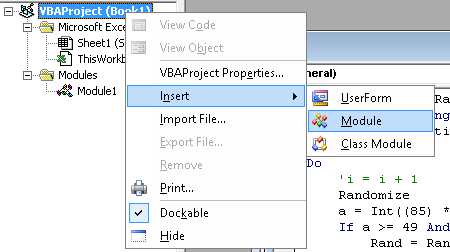## Posts

Showing posts from December, 2015

### Random numberFor any reason, if you need to generate a alphanumeric password, the VBA code below will be able to help you. 1. Press Alt+F11 to bring up the Visual Basic Editor. 2. Right click the book object and select Insert > Module. 3. Paste the code below into the Module window. 4. At any cell enter function =RandomizeF() 5. This code will skip Capital "I", Capital "O", small letter "l", small letter "o" because I use this code to generate random password. In order to avoid confuse, I decide to remove these letter. * Public Function RandomizeF() Dim Rand As String Application.Volatile Do     'i = i + 1     Randomize     a = Int((85) * Rnd + 38)     If a >= 49 And a <= 57 Then         Rand = Rand & Chr(a)     ElseIf a >= 65 And a <= 72 Then         Rand = Rand & Chr(a)     ElseIf a >= 74 And a <= 78 Then         Rand = Rand & Chr(a)     ElseIf a >= 80 And a <= 90 Then         R